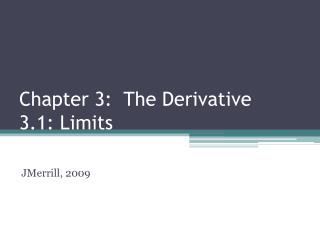DownloadDownload PresentationChapter 3: The Derivative 3.1: Limits

# Chapter 3: The Derivative 3.1: Limits

Télécharger la présentation## Chapter 3: The Derivative 3.1: Limits

- - - - - - - - - - - - - - - - - - - - - - - - - - - E N D - - - - - - - - - - - - - - - - - - - - - - - - - - -
##### Presentation Transcript

1. Chapter 3: The Derivative3.1: Limits JMerrill, 2009

2. Algebra deals with static situations (what will something cost in 3 years?) • Calculus deals with dynamic situations (when does the growth of a population begin to slow down) • The concept of a limit is a fundamental concept in calculus. One use is in applications that include maximizations. • The idea of the limit of a function is what connects algebra and geometry to the mathematics of calculus.

3. There are multiple methods to Finding Limits: Case 1 • If a polynomial function is defined at a point, then the limit is obtained by direct substitution • Example: • You Do: 10

4. Case 2 • In a rational function, if direct substitution results in a 0 in both the numerator and denominator, (this is called the indeterminant form) factor both, reduce to lowest terms, then use direct substitution. • Example:

5. Case 3 • In a rational function, if direct substitution results in division by zero, evaluate small increments close to a, from both the left and the right. Note that x = a is a vertical asymptote. In order for a function to have a limit at a point, its right- and left-hand limits have to be the same. If not, the limit does not exist. The limit may occur at the vertical asymptote.

6. Example

7. Example

8. Definition of a Limit • If f(x) becomes arbitrarily close to a unique number L as x approaches c from either side, the limit of f(x) as x approaches c is L. This is written

9. Case 4 • You can estimate the limit by substituting increasingly larger values for n. (look at 100, 1000, 100000000…) • Ex: Find . When n = 100, • As n gets larger and larger, approaches 0 and so does . So, = 0.

10. Case 4 Continued • Ex: Find : (0.99)1000 = 4.3 x 10-5. (0.99)10,000 = 2.2 x 10-44 • So,

11. Case 5 • Divide the numerator and denominator by the highest power of n that occurs in the denominator.

12. Example What is the highest power of n in the denominator?

13. You Do

14. Increasing Without Bound • Sometimes, the terms of a sequence increase without bound, in which case, the limit does not exist. • Ex: 3, 7, 11, 15, … 4n – 1, … so • Ex: -10, -100, -1000, … -10n, … so

15. You Do Therefore, the limit DNE